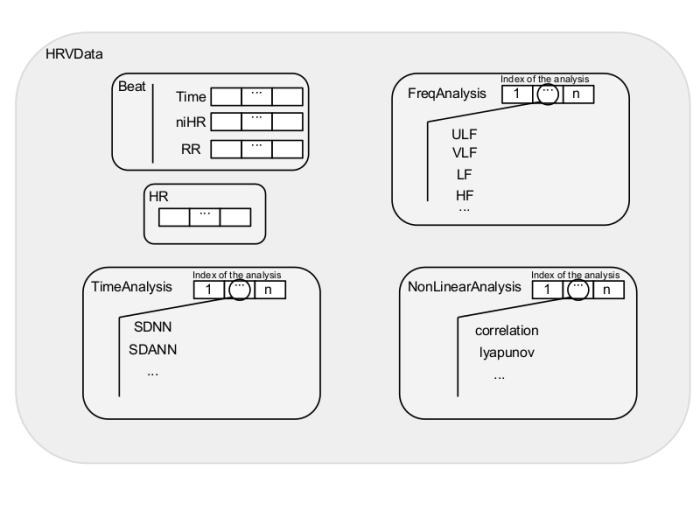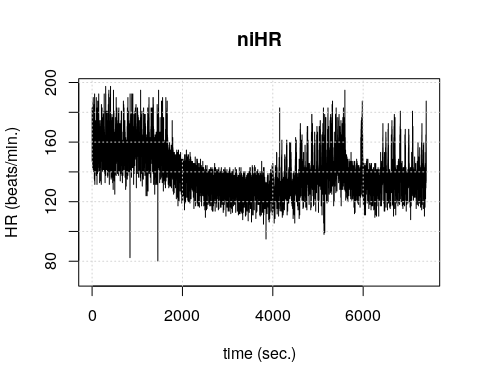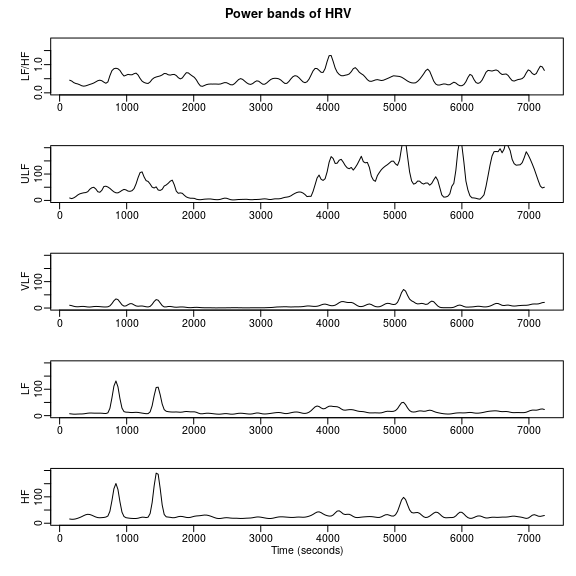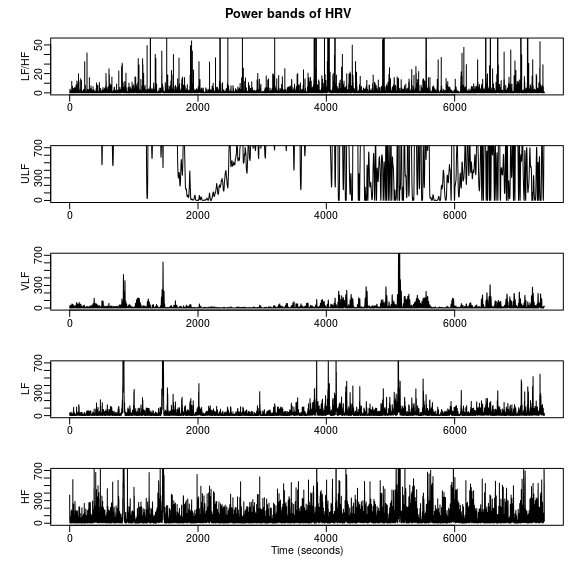# RHRV Quick Start Tutorial

#### 2019-10-30

In this vignette, a brief description of the RHRV package is presented. Due to the large collection of features that RHRV offers, we shall only refer to the most important functionality to perform a basic Heart Rate Variability (HRV) analysis. The interested reader is referred to the free tutorial for further information.

## Installation

This vignette assumes that the user has some basic knowledge of the R environment. If this is not your case, you can find a nice introduction to R in the R project homepage. The R project homepage also provides an R Installation and Administration guide. Once you have download and installed R, you can install RHRV by typing:

install.packages("RHRV", dependencies = TRUE)

You can also install it by downloading it from the CRAN. Once the download has finished, open R, move to the directory where you have download it (by using the R command setwd and type:

setwd(sourceDirectory)
install.packages("RHRV_XXX",repos = NULL)

Here, XXX is the version number of the library. To start using the library, you should load it by using:

library('RHRV')

## A 15-minutes guide to RHRV

We propose the following basic program flow to perform a basic HRV analysis using the RHRV package:

• Load heart beat positions. For the sake of simplicity, in this document we will focus in ASCII files.
• Build the instantaneous Heart Rate (HR) series and filter it to eliminate spurious points.
• Plot the instantaneous HR series.
• Interpolate the instantaneous HR series to obtain a HR series with equally spaced values.
• Plot the interpolated HR series.
• Perform the desired analysis. The user can perform time-domain analysis, frequency-domain analysis and/or nonlinear analysis. Since nonlinear analysis techniques make use of advanced concepts, this vignette focuses in time and frequency domain analysis.
• Plot the results of the analysis that has been performed.

We shall deal with each of these points in the following sections. All the examples of this vignette will use the example beats file “example.beats” that may be downloaded from here. Additionally, the data from this file has been included in RHRV. The user can access this data executing:

# HRVData structure containing the heart beats
data("HRVData")
# HRVData structure storing the results of processing the
# heart beats: the beats have been filtered, interpolated, ...
data("HRVProcessedData")

The example file is an ASCII file that contains the beats positions obtained from a 2 hours electrocardiogram (ECG), with one beat position per row. The subject of the ECG is a patient suffering from paraplegia and hypertension (systolic blood pressure above 200 mm-Hg). During the recording, he is supplied with prostaglandin E1 (a vasodilator that is rarely employed) and systolic blood pressure fell to 100 mm-Hg for over an hour. Then, the blood pressure increased slowly up to approximately 150 mm-Hg.

## Preprocessing the Heart Rate series

RHRV uses a custom data structure called HRVData to store all HRV information related to the signal being analysed. The following Figure summarizes the most important fields in the HRVData structure. HRVData is implemented as a list object in R language. This list contains all the information corresponding to the signal to be analysed, some parameters generated by the pre-processing functions and the HRV analysis results. It must be noted that, since the HRVData structure is a list, each of its fields can be accessed using the $ operator of the R language.The most important fields stored in the HRVData structure hrv.data = CreateHRVData() hrv.data = SetVerbose(hrv.data, TRUE ) After creating the empty HRVData structure the next step should be loading the signal that we want to analyse into this structure. RHRV imports data files containing heart beats positions. Supported formats include ASCII (LoadBeatAscii function), EDF (LoadBeatEDFPlus), Polar (LoadBeatPolar), Suunto (LoadBeatSuunto) and WFDB data files (LoadBeatWFDB). For the sake of simplicity, we will focus in ASCII files containing one heart beat occurrence time per line. We also assume that the beat occurrence time is specified in seconds. For example, let’s try to load the “example.beats” file, whose first lines are shown below. Each line denotes the occurrence time of each heartbeat. 0 0.3280001 0.7159996 1.124 1.5 1.88 ... In order to load this file, we may write: hrv.data = LoadBeatAscii(hrv.data, "example.beats", RecordPath = "beatsFolder") ## Loading beats positions for record: example.beats ## Path: beatsFolder ## Scale: 1 ## Date: 01 / 01 / 1900 ## Time: 00 : 00 : 00 ## Number of beats: 17360 The console information is only displayed if the verbose mode is on. The Scale parameter is related to the time units of the file. 1 denotes seconds, 0.1 tenth of seconds and so on. The Date and Time parameters specify when the file was recorded. The RecordPath can be omitted if the RecordName is in the working directory. Further information about this function and other input formats may be found in the online tutorial. ### Calculating HR and filtering To compute the HRV time series the BuildNIHR function can be used (Build Non Interpolated Heart Rate). This function constructs both the RR and instantaneous heart rate (HR) series. We will refer to the instantaneous Heart Rate as the niHR (non interpolated Heart Rate) series. Both series are stored in the HRVData structure. hrv.data = BuildNIHR(hrv.data) ## Calculating non-interpolated heart rate ## Number of beats: 17360 A Filtering operation must be carried out in order to eliminate outliers or spurious points present in the niHR time series with unacceptable physiological values. Outliers present in the series originate both from detecting an artefact as a heartbeat (RR interval too short) or not detecting a heartbeat (RR interval too large). The outliers removal may be both manual or automatic. In this quick introduction, we will use the automatic removal. The automatic removal of spurious points can be performed by the FilterNIHR function. The FilterNIHR function also eliminates points with unacceptable physiological values. hrv.data = FilterNIHR(hrv.data) ## Filtering non-interpolated Heart Rate ## Number of original beats: 17360 ## Number of accepted beats: 17248 ### Interpolating In order to be able to perform spectral analysis in the frequency domain, a uniformly sampled HR series is required. It may be constructed from the niHR series by using the InterpolateNIHR function, which uses linear (default) or spline interpolation. The frequency of interpolation may be specified. 4 Hz (the default value) is enough for most applications. # Note that it is not necessary to specify freqhr since it matches with # the default value: 4 Hz hrv.data = InterpolateNIHR(hrv.data, freqhr = 4) ## Interpolating instantaneous heart rate ## Frequency: 4 Hz ## Number of beats: 17248 ## Number of points: 29592 ### Plotting Before applying the different analysis techniques that RHRV provides, it is usually interesting to plot the time series with which we are working. The PlotNIHR function permits the graphical representation of the niHR series whereas the PlotHR function permits to graphically represent the interpolated HR time series. The two plots are usually very similar. For example, we could type: PlotNIHR(hrv.data, main = "niHR") ## Plotting non-interpolated instantaneous heart rate ## Number of points: 17248As seen in the previous figure, the patient initially had a heart rate of approximately 160 beats per minute. Approximately half an hour into record the prostaglandina E1 was provided, resulting in a drop in heart rate to about 130 beats per minute during about 40 minutes, followed by a slight increase in heart rate. ## Analysing the Heart Rate series ### Time-domain analysis techniques The simplest way of performing a HRV analysis in RHRV is using the time analysis techniques provided by the CreateTimeAnalysis function. This function computes the most widely used time-domain parameters and stores them in the HRVData structure. The most interesting parameter that the user may specify is the width of the window that will be used to analyse short segments from the RR time series (size parameter, in seconds). Concretely, several statistics will be computed for each window. By studying how these statistics evolve through the recording, a set of time parameters will be computed (For example, the SDANN and SDNNIDX parameters). Other important argument that can be tuned is the interval width of the bins that will be used to compute the histogram (interval parameter). As an alternative to the interval parameter, the user may use the numofbins parameter to specify the number of bins in the histogram. A typical value for the size parameter is 300 seconds (which is also the default value), whereas that a typical value for the interval is about 7.8 milliseconds (also default value). hrv.data = CreateTimeAnalysis(hrv.data, size = 300, interval = 7.8125) If the verbose mode is on, the program will display the results of the calculations on the screen. Otherwise, the user must access the “raw” data using the $ operator of the R language.

Finally, we show a complete example for performing a basic time-domain analysis. The console output is also shown. It should be noted that it is not necessary to perform the interpolation process before applying the time-domain techniques since these parameters are calculated directly from the non interpolated RR-time series.

hrv.data = CreateHRVData()
hrv.data = SetVerbose(hrv.data,FALSE)
hrv.data = BuildNIHR(hrv.data)
hrv.data = FilterNIHR(hrv.data)
hrv.data = SetVerbose(hrv.data,TRUE)
hrv.data = CreateTimeAnalysis(hrv.data,size=300,interval = 7.8125)
##  Creating time analysis
##  Size of window: 300 seconds
##  Width of bins in histogram: 7.8125 milliseconds
##  Number of windows: 24
##  Data has now 1 time analyses
##    SDNN: 39.7449 msec.
##    SDANN: 31.1059 msec.
##    SDNNIDX: 24.9566 msec.
##    pNN50: 9.306 %
##    SDSD: 30.8642 msec.
##    r-MSSD: 30.8633 msec.
##    IRRR: 56 msec.
##    TINN: 172.0945 msec.
##    HRV index: 11.014
# We can access "raw" data... let's print separately, the SDNN
# parameter
cat("The SDNN has a value of ",hrv.data$TimeAnalysis[]$SDNN," msec.\n")
## The SDNN has a value of  39.74489  msec.

### Frequency-domain analysis techniques

A major part of the functionality of the RHRV package is dedicated to the spectral analysis of HR signals. Before performing the frequency analysis, a data analysis structure must be created. Such structure shall store the information extracted from a variability analysis of the HR signal as a member of the FreqAnalysis list, under the HRVData structure. Each analysis structure created is identified by a unique number (in order of creation). To create such an analysis structure, the CreateFreqAnalysis function is used.

hrv.data = CreateFreqAnalysis(hrv.data)
##  Creating frequency analysis
##  Data has now 1 frequency analysis

Notice that, if verbose mode is on, the CreateFreqAnalysis function informs us about the number of frequency analysis structures that have been created. In order to select a particular spectral analysis, we will use the indexFreqAnalysis parameter in the frequency analysis functions.

The most important function to perform spectral HRV analysis is the CalculatePowerBand function. The CalculatePowerBand function computes the spectrogram of the HR series in the ULF (Ultra Low Frequency), VLF (Very Low Frequency), LF (Low Frequency) and HF (High Frequency) bands using the Short Time Fourier Transform (STFT) or wavelets. Boundaries of the bands may be chosen by the user. If boundaries are not specified, default values are used: ULF, [0, 0.03] Hz; VLF, [0.03, 0.05] Hz; LF, [0.05, 0.15] Hz; HF, [0.15, 0.4] Hz. The type of analysis can be selected by the user by specifying the type parameter of the CalculatePowerBand function. The possible options are either "fourier" or "wavelet". Because of the backwards compatibility, the default value for this parameter is "fourier".

#### Fourier

When using the STFT to compute the spectrogram using the CalculatePowerBand function, the user may specify the following parameters related with the STFT:

• Size: the size of window for calculating the spectrogram measured in seconds. The RHRV package employs a Hamming window to perform the STFT.
• Shift: the displacement of window for calculating the spectrogram measured in seconds.
• Sizesp: the number of points for calculating each window of the STFT. If the user does not specify it, the program selects a proper length for the calculations (it selects sizesp so that sizesp$$=2^m$$, for some $$m \in \mathbb{N}$$).

When using CalculatePowerBand, the indexFreqAnalysis parameter (in order to indicate which spectral analysis we are working with) and the boundaries of the frequency bands may also be specified.

As an example, let’s perform a frequency analysis in the typical HRV spectral bands based on the STFT . We may select 300 s (5 minutes) and 30 s as window size and displacement values because these are typical values when performing HRV spectral analysis. We let the program choose the value of the zero-padding. Thus, we may write:

hrv.data = CreateHRVData( )
hrv.data = SetVerbose(hrv.data,FALSE)
hrv.data = BuildNIHR(hrv.data)
hrv.data = FilterNIHR(hrv.data)
hrv.data = InterpolateNIHR (hrv.data, freqhr = 4)
hrv.data = CreateFreqAnalysis(hrv.data)
hrv.data = SetVerbose(hrv.data,TRUE)
# Note that it is not necessary to write the boundaries
# for the frequency bands, since they match
# the default values
hrv.data =
CalculatePowerBand(hrv.data , indexFreqAnalysis = 1,
size = 300, shift = 30, type = "fourier",
ULFmin = 0, ULFmax = 0.03, VLFmin = 0.03, VLFmax = 0.05,
LFmin = 0.05, LFmax = 0.15, HFmin = 0.15, HFmax = 0.4 )
##  Calculating power per band
##  Using Fourier analysis
##  Windowing signal... 237 windows
##  Power per band calculated

Alternatively, since most values of the arguments match its default values we could have written:

hrv.data = CalculatePowerBand(hrv.data, indexFreqAnalysis= 1,
size = 300, shift = 30)

#### Wavelets

When using Wavelet analysis with the CalculatePowerBand function, the user may specify:

• wavelet: mother wavelet used to calculate the spectrogram. Some of the most widely used Wavelets are available: Haar ("haar"), extremal phase ("d4", "d6", "d8" and "d16") and the least asymmetric Daubechies ("la8", "la16" and "la20") and the best localized Daubechies ("bl14" and "bl20") Wavelets among others. The default value is "d4". The name of the wavelet specifies the family (the family determines the shape of the Wavelet and its properties) and the length of the wavelet. For example, "la8" belongs to the Least Asymmetric family and has a length of 8 samples. We may give a simple advice for wavelet selection based on the wavelet’s length: shorter wavelets usually have better temporal resolution, but worse frequency resolution. On the other hand, longer wavelets usually have worse temporal resolution, but they provide better frequency resolution. Better temporal resolution means that we can study shorter time intervals. On the other hand, a better frequency resolution means better frequency discrimination. That is, shorter wavelets will tend to fail when discriminating close frequencies.

• bandtolerance: maximum error allowed when the Wavelet-based analysis is performed. It can be specified as an absolute or a relative error depending on the relative parameter value. Default value is 0.01.
• relative: logic value specifying which type of band tolerance shall be used: relative (in percentage) or absolute (default value). For the sake of simplicity, in this document we will use the absolute band tolerance.

Let’s analyse the same frequency bands as before but using the wavelet-algorithm. For the sake of simplicity, we will use an absolute tolerance of 0.01 Hz. We may select the least asymmetric Daubechies of width 8 ("la8") as wavelet, since it provides a good compromise between frequency and time resolution. Thus, we may write:

hrv.data = CreateHRVData( )
hrv.data = SetVerbose(hrv.data,FALSE)
hrv.data = BuildNIHR(hrv.data)
hrv.data = FilterNIHR(hrv.data)
hrv.data = InterpolateNIHR (hrv.data, freqhr = 4)
hrv.data = CreateFreqAnalysis(hrv.data)
hrv.data = SetVerbose(hrv.data,TRUE)
# Note that it is not necessary to write the boundaries
# for the frequency bands, since they match the default values
hrv.data =
CalculatePowerBand( hrv.data , indexFreqAnalysis = 1,
type = "wavelet", wavelet = "la8",
bandtolerance = 0.01, relative = FALSE,
ULFmin = 0, ULFmax = 0.03, VLFmin = 0.03, VLFmax = 0.05,
LFmin = 0.05, LFmax = 0.15, HFmin = 0.15, HFmax = 0.4 )
##  Calculating power per band
##  Using Wavelet analysis
##  Power per band calculated

#### Creating several analyses

In the previous examples we have used just one frequency analysis to illustrate the basic use of CalculatePowerBand. However, it is possible to create and use the same HRVData for performing several spectral analysis. When we do this, we use the parameter indexFreqAnalysis to indicate which spectral analysis we are working with. For example, we could perform both Fourier and wavelet based analysis:

# ...
# create structure, load beats, filter and interpolate
hrv.data = CreateFreqAnalysis(hrv.data)
hrv.data = SetVerbose(hrv.data, FALSE)
# use freqAnalysis number 1 for perfoming
# Fourier analysis. This time, we do not
# write the band's boundaries
hrv.data = CalculatePowerBand(hrv.data , indexFreqAnalysis = 1,
size = 300, shift = 30, sizesp = 2048,
type = "fourier")
# use freqAnalysis number 2 for perfoming
# wavelet analysis. Note the indexFreqAnalysis = 2!!!
hrv.data = CreateFreqAnalysis(hrv.data)
hrv.data = CalculatePowerBand(hrv.data, indexFreqAnalysis= 2,
type = "wavelet", wavelet = "la8",
bandtolerance = 0.01, relative = FALSE)

#### Plotting

RHRV also includes plotting utilities for representing the spectrogram of each frequency band: the PlotPowerBand function. PlotPowerBand receives as inputs the HRVData structure and the index of the frequency analysis that the user wants to plot (indexFreqAnalysis argument). Optionally, the user can specify additional parameters for modifying the plots (whether to use or not normalized plots, specify the y-axis, etc.). For the sake of simplicity we will only use the ymax parameter (for specifying the maximum y-axis of the power bands plot) and the ymaxratio parameter (for specifying the maximum y-axis in the LF/HF plot).

If we want to plot the power bands computed in the previous example, we may use:

# Plotting Fourier analysis
PlotPowerBand(hrv.data, indexFreqAnalysis = 1, ymax = 200, ymaxratio = 1.7)# Plotting wavelet analysis
PlotPowerBand(hrv.data, indexFreqAnalysis = 2, ymax = 700, ymaxratio = 50)#### A brief comparison: Wavelets Vs. Fourier

The previous Figures illustrate some of the most important differences between Fourier and wavelet-based analysis. The most important differences may be summarized as follows:

• The power range is not the same when using Fourier than when using wavelets due to the windowing used in both techniques. Thus, we should avoid direct comparisons between the numerical results obtained with Fourier with those obtained using wavelets.
• The Fourier’s power spectrum is smoother than the wavelet’s power spectrum. This is a consequence of the higher temporal resolution that the wavelet-based analysis provides. We could try to increase Fourier’s frequency resolution by decreasing the window’ size used in the analysis. The shorter window we use, the sharper spectrum we get. Similarly, we can increase/decrease temporal resolution using shorter/larger wavelets when performing wavelet-based analysis.
• The power spectrum obtained from the Fourier-based analysis has a smaller number of samples than the original signal as a consequence of the use of windows. Conversely, the power spectrum obtained from the wavelet-based analysis has the same number of samples as the original RR time series.